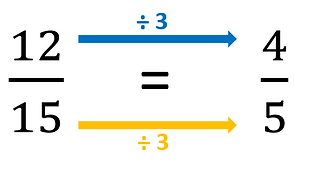top of page

## Topic 13: Equivalent fractionsFractions can always be expressed in more than one way.

Here are several ways of expressing the fraction 1/2.

These different versions of the same fractions are called equivalents

In your exam, you may be shown a fraction and asked to give an equivalent.

For example, you may be asked for a fraction equivalent to 1/4.

An easy way to find an equivalent is to double both the numerator and the denominator. This gives 2/8.  1/4 = 2/8.

If you multiply or divide both numerator and denominator by the same amount, you will arrive at an equivalent.

Simplifying fractions

Sometimes you will be asked to give a fraction in its simplest form (also called the lowest terms).

In this case, you need to divide the numerator and the denominator by the same amount.

For example, you may be asked for the simplest version of 2/10.

Both numerator and denominator will divide by 2, giving an equivalent of 1/5.

A useful method for checking if a fraction can be simplified is the '2, 3, 5' method. It doesn't work for all fractions, but it will usually work for Level 1 exam questions.

When asked to simplify a fraction, see if both numerator and denominator will divide by 2. If so, you can easily simplify the fraction:

If both numbers will not divide by 2, see if they will divide by 3:

If both numbers will not divide by 2 or 3, see if they will divide by 5:

Using this method, you may need to simplify several times to arrive at the simplest form.bottom of page